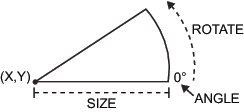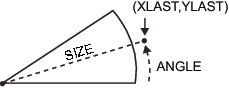# PIEXY Function

Calculates a point on the outline of the slice arc.

## Syntax

FUNCTION='PIEXY';

### Associated Variables

ANGLE=rotation-angle
specifies the angle of rotation when moving around the perimeter of a pie. The ANGLE variable determines the angle at which the point is located relative to 0 (the three o'clock position). The default is 0.00.
determines the distance from the center of the slice to the point that is being calculated. The point's distance is the current value of the SIZE variable multiplied by the radius (that is, the SIZE variable) of the previously drawn slice. To position a graphics element inside the pie slice, set the SIZE variable to less than 1; to position it outside of the pie slice, set the SIZE variable to greater than 1. For example, if you specify these statements, the point calculated is 1.1 times the radius (where the radius is taken from the SIZE variable that is used with the previous FUNCTION='PIE' or FUNCTION='PIECNTR' observation).
`function="piexy"; size=1.1; output;`
WHEN='B' | 'A'
specifies when to update the internal coordinate pair (XLAST, YLAST) in relation to other procedure output. See

## Details

PIEXY does not draw anything but places the calculated coordinates of the point in the internal coordinate pair (XLAST, YLAST). Then you can use XLAST and YLAST with other functions to perform other graphics actions, such as labeling pie slices. If you need to use the calculated position for a text function, use the SWAP or CNTL2TXT to put (XLAST, YLAST) into (XLSTT, YLSTT).
PIEXY assumes that a pie slice has been drawn or that FUNCTION='PIECNTR' has been used. Erroneous results can occur if a slice has not been drawn and PIEXY is invoked.
Pie Slice Drawn with the PIE Function shows a pie slice that is drawn with the PIE function. Point Calculated with the PIEXY Function shows a point beyond the arc that was calculated using the PIEXY function.
Pie Slice Drawn with the PIE FunctionPoint Calculated with the PIEXY Function